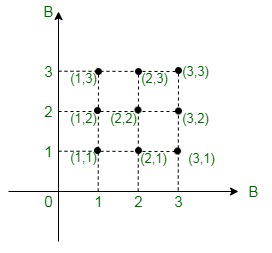Open In App
Related Articles
• RD Sharma Class 11 Solutions for Maths

# Class 11 RD Sharma Solutions – Chapter 2 Relations – Exercise 2.1

### Question 1(i). If (a/3 + 1, b – 2/3) = (5/3, 1/3), find the values of a and b.

Solution:

According to the definition of equality of ordered pairs

(a/3 + 1, b – 2/3) = (5/3, 1/3)

⇒ a/3 + 1 = 5/3 and b – 2/3 =1/3

⇒ a/3 = (5 – 3)/3 and b = (1/3 + 2/3)

⇒ a/3 = 2/3 and b = 3/3

⇒ a = 2 and b = 1

### Question 1(ii). If (x + 1, 1) = (3, y – 2), find the values of x and y.

Solution:

According to the definition of equality of ordered pairs

(x + 1, 1) = (3, y – 2)

⇒ x + 1 = 3 and 1 = y – 2

⇒ x = 3 – 1 and 1 + 2 = y

⇒ x = 2 and 3 = y

⇒ x = 2 and y = 3

### Question 2. If the ordered pairs (x, -1) and (5, y) belong to the set {(a, b) : b = 2a – 3}, find the values of x and y.

Solution:

Given:

(x, -1) ∈ {(a, b) : b = 2a – 3}

and, (5, y) ∈ {(a, b) : b = 2a – 3}

⇒ -1 = 2 × x – 3 and y = 2 × 5 – 3

⇒ -1 = 2x – 3 and y = 10 – 3

⇒ 3 – 1 = 2x and y = 7

⇒ 2 = 2x and y = 7

⇒ x = 1 and y = 7

### Question 3. If a ∈ {- 1, 2, 3, 4, 5} and b ∈ {0, 3, 6}, write the set of all ordered pairs (a, b) such that a + b = 5.

Solution:

Given: a ∈ {- 1, 2, 3, 4, 5} and b ∈ {0, 3, 6},

Now, we have to find the ordered pair (a, b) such that a + b = 5

So, the ordered pair (a, b) such that a + b = 5 are as follows

(a, b) ∈ {(- 1, 6), (2, 3), (5, 0)}

### Question 4. If a ∈ {2, 4, 6, 9} and b ∈ {4, 6, 18, 27}, then form the set of all ordered pairs (a, b) such that a divides b and a<b.

Solution:

Given: a ∈ {2, 4, 6, 9} and b ∈ {4, 6, 18, 27}

Here,

2 divides 4, 6, 18 and is also less than all of them

4 divides 4 and is also less than none of them

6 divides 6, 18 and is less than 18 only

9 divides 18, 27 and is less than all of them

∴ Ordered pairs (a, b) are (2, 4), (2, 6), (2, 18), (6, 18), (9, 18) and (9, 27)

### Question 5. If A = {1, 2} and B = {1, 3}, find A x B and B x A.

Solution:

Given: A = {1, 2} and B = {1, 3}

Now we have to find A x B, and B x A

A × B = {1, 2} × {1, 3}

= {(1, 1), (1, 3), (2, 1), (2, 3)}

B × A = {1, 3} × {1, 2}

= {(1, 1), (1, 2), (3, 1), (3, 2)}

### Question 6. Let A = {1, 2, 3} and B = {3, 4}. Find A x B and show it graphically

Solution:

Given: A = {1, 2, 3} and B = {3, 4}

Now we have to find A x B

A x B = {1, 2, 3} × {3, 4}

= {(1, 3), (1, 4), (2, 3), (2, 4), (3, 3), (3, 4)}

To draw A x B graphically follow the following steps:

Step 1: Draw horizontal and vertical axis.

Step 2: The horizontal axis represents set A and the vertical axis represents set B.

Step 3: Now, draw dotted lines perpendicular to horizontal and vertical axes through the elements of set A and B

Step 4: Point of intersection of these perpendicular represents A × B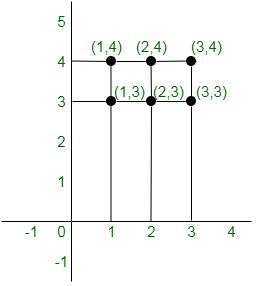### Question 7. If A = {1, 2, 3} and B = {2, 4}, what are A x B, B x A, A x A, B x B, and (A x B) ∩ (B x A)?

Solution:

Given:

A = {1, 2, 3} and B = {2, 4}

Now we have to find A × B, B × A, A × A, and (A × B) ∩ (B × A)

A × B = {1, 2, 3} × {2, 4}

= {(1, 2), (1, 4), (2, 2), (2, 4), (3, 2), (3, 4)}

B × A = {2, 4} × {1, 2, 3}

= {(2, 1), (2, 2), (2, 3), (4, 1), (4, 2), (4, 3)}

A × A = {1, 2, 3} × {1, 2, 3}

= {(1, 1), (1, 2), (1, 3), (2, 1), (2, 2), (2, 3), (3, 1), (3, 2), (3, 3)}

B × B = {2, 4} × {2, 4}

= {(2, 2), (2, 4), (4, 2), (4, 4)}

Intersection of two sets represents common elements of both the sets

So,

(A × B) ∩ (B × A) = {(2, 2)}

### Question 8. If A and B two sets having 3 elements in common. If n(A) = 5, n(B) = 4, Find n(A × B) and n[(A × B) ∩ (B × A)]

Solution:

Given: n(A) = 5 and n(B) = 4

We know that if A and B are two finite sets, then n(A × B) = n(A) × n(B)

Therefore,

n(A × B) = 5 × 4 = 20

Now,

n[(A × B) ∩ (B × A)] = 3 × 3 = 9          -(∵ A and B have 3 common elements)

### Question 9. Let A and B two sets. Show that the sets A × B and B × A has an elements in common if the sets A and B have an element in common.

Solution:

Let us considered(a, b) be an arbitrary elements of (A × B) ∩ (B × A). Then,

(a, b) ∈ (A × B) ∩ (B × A)

= (a, b) ∈ A × B and (a, b) ∈ B × A

= (a ∈ A and b ∈ B) and (a ∈ B and b ∈ A)

= (a ∈ A and a ∈ B) and (b ∈ A and b ∈ B)

= a ∈ A ∩ B and b ∈ A ∩ B

Hence, the sets A × B and B × A have an element in

common have an element in common.

### Question 10. Let A and B two sets such that n(A) = 3 and n(B) = 2. If (x, 1), (y, 2), (z, 1) are in A × B, find A and B where x, y, z are distinct elements

Solution:

Since (x, 1), (y, 2), (z, 1) are elements of A × B. Therefore, x, y, z ∈ A and 1, 2 ∈ B

Given: n(A) = 3 and n(B) = 2

Therefore, x, y, z ∈ A and n(A) = 3

⇒ A = (x, y, z)

1, 2 ∈ B and n(B) = 2

⇒ B = (1, 2)

### Question 11. Let A = {1, 2, 3, 4} and R = {(a, b) : a ∈ A, b ∈ A, a divides b}. Write R explicitly.

Solution:

Given: A = (1, 2, 3, 4) and, R = {(a, b) : a ∈ A, b ∈ A, a divides b}

Now, a/b stands for ‘a divides b’.

So, for the elements of the given sets, we find that 1/1, 1/2, 1/3, 1/4, 2/2, 3/3 and 4/4

Therefore,

R = {(1, 1), (1, 2), (1, 3), (1, 4), (2, 2), (2, 4), (3, 3), (4, 4)}

### Question 12. If A = {-1, 1}, find A × A × A

Solution:

Given: A = {-1, 1}

So, A × A = {-1, 1} × {-1, 1}

= {(-1, -1), (-1, 1), (1, -1), (1, 1)}

Therefore, A × A × A = {-1, 1} × {(-1, -1), (-1, 1), (1, -1)(1, 1)}

= {(-1, -1, -1), (-1, -1, 1), (-1, 1, -1), (-1, 1, 1), (1, -1, -1), (1, -1, 1), (1, 1, -1), (1, 1, 1)}

### Question 13. State whether each of the following statements are true or false. If the statements is false, re-write the given statement correctly:

(i) If P = {m, n} and Q = {n, m}, then P × Q = {(m, n), (n, m)}

(ii) If A and B are non-empty sets, then A × B is a non-empty set of ordered pairs (x, y) such that x ∈ B and y ∈ A.

(iii) If A = {1, 2} and B = {3, 4}, then A ∩ (B ∩ ∅) = ∅

Solution:

(i) False,

If P = {m, n} and Q = {n, m},

Then,

P × Q = {(m, n), (m, m), (n, n), (n, m)}

(ii) False,

If A and B are non-empty sets, then AB is a non-empty set of ordered pairs (x, y) such that x ∈ A and y ∈ B

(iii) True

### Question 14. If A = {1, 2} form the set A × A × A

Solution:

Given: A = {1, 2}

So, A × A = {1, 2} × {1, 2}

= {(1, 1), (1, 2), (2, 1), (2, 2)}

Therefore,

A × A × A = {1, 2} × {(1, 1), (1, 2), (2, 1), (2, 2)}

= {(1, 1, 1), (1, 1, 2), (1, 2, 1), (1, 2, 2), (2, 1, 1), (2, 1, 2), (2, 2, 1), (2, 2, 2)}

### Question 15 (i). If A = {1, 2, 4} and B = {1, 2, 3}, represent A × B graphically

Solution:

Given: A = {1, 2, 4} and B = {1, 2, 3}

So, A × B = {1, 2, 4} × {1, 2, 3}

= {(1, 1), (1, 2), (1, 3), (2, 1), (2, 2), (2, 3), (4, 1), (4, 2), (4, 3)}

Hence, we represent A on the horizontal line and B on vertical line.

So, the graphical representation of A × B is as shown below: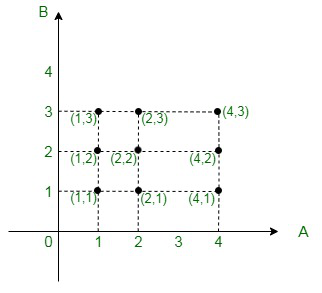### Question 15 (ii). If A = {1, 2, 4} and B = {1, 2, 3}, represent B × A graphically.

Solution:

Given: A = {1, 2, 4} and B = {1, 2, 3}

So, B × A = {1, 2, 3} × {1, 2, 4}

= {(1, 1), (1, 2), (1, 4), (2, 1), (2, 2), (2, 4), (3, 1), (3, 2), (3, 4)}

Hence, we represent B on the horizontal line and A on vertical line.

So, the graphical representation of B × A: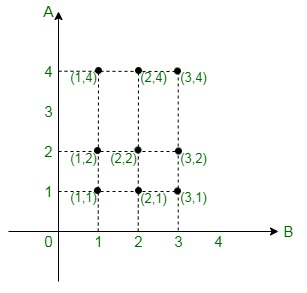### Question 15 (iii). If A = {1, 2, 4} and B = {1, 2, 3}, represent A × A graphically.

Solution:

Given: A = {1, 2, 4}, B = {1, 2, 3}

So, A × A = {1, 2, 4} × {1, 2, 4}

= {(1, 1), (1, 2), (1, 4), (2, 1), (2, 2), (2, 4), (4, 1), (4, 2), (4, 4)}

Graphical representation of A × A: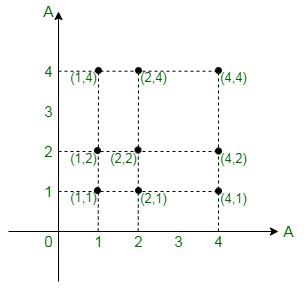### Question 15 (iv). If A = {1, 2, 4} and B = {1, 2, 3}, represent B × B graphically.

Solution:

Given: A = {1, 2, 4}, B = {1, 2, 3}

So, B × B = {1, 2, 3} × {1, 2, 3}

= {(1, 1), (1, 2), (1, 4), (2, 1), (2, 2), (2, 3), (3, 1), (3, 2), (3, 3)}

Graphical representation of B × B: